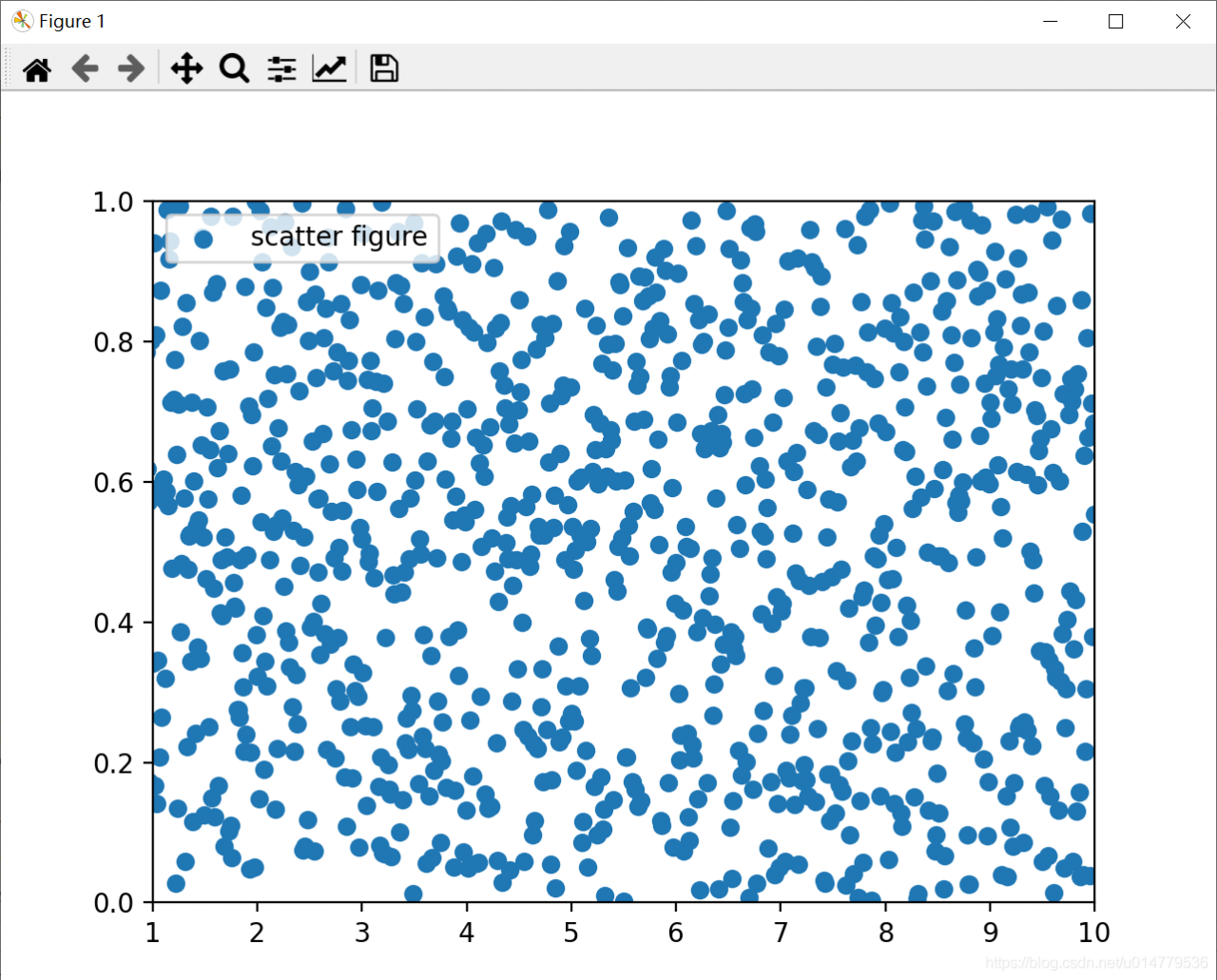# Matplotlib of data visualization plt.xlim The () ylim() function sets the x-axis and y-axis range coordinates

``````import matplotlib.pyplot as plt
import numpy as np

x = np.linspace(0.05,10,1000)
y = np.random.rand(1000)

plt.scatter(x,y,label="scatter figure")

plt.legend()

plt.xlim(1,10)
plt.ylim(0,1)

plt.show()
``````